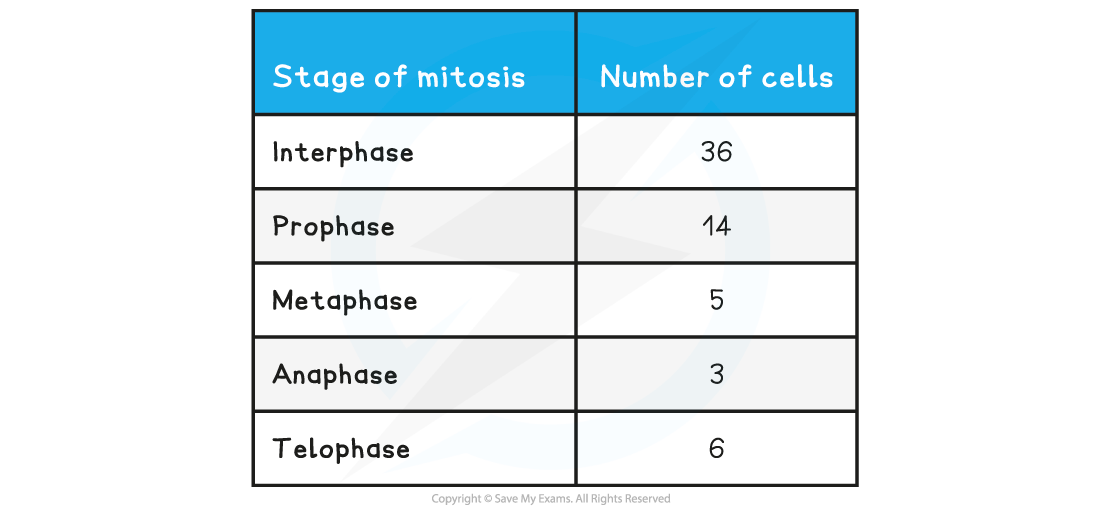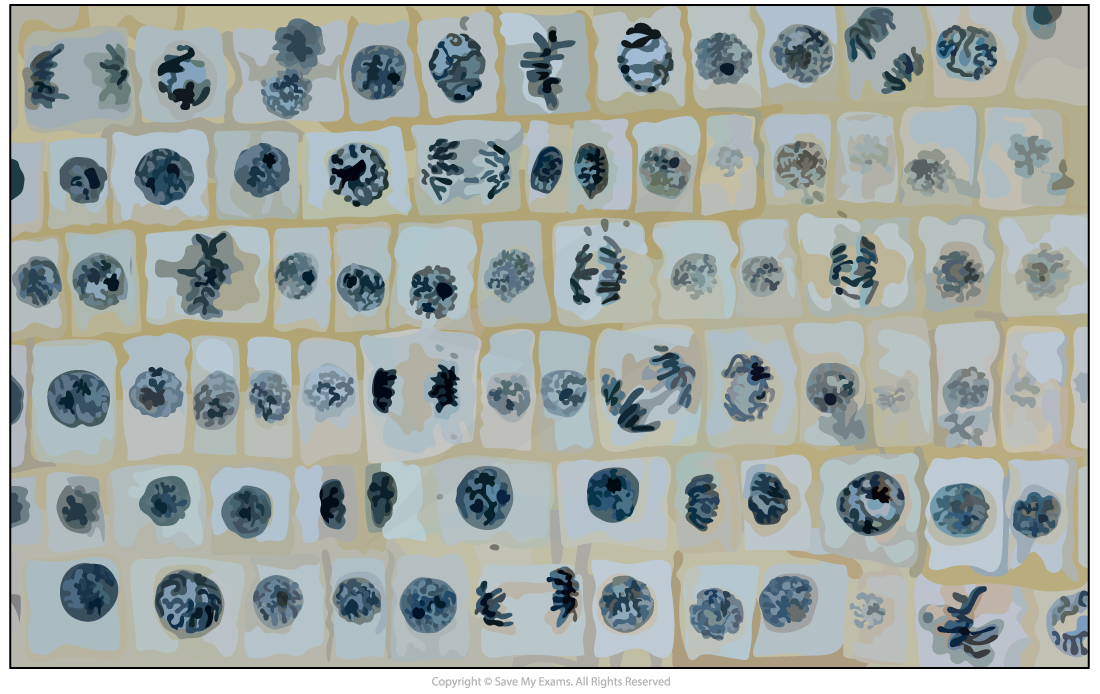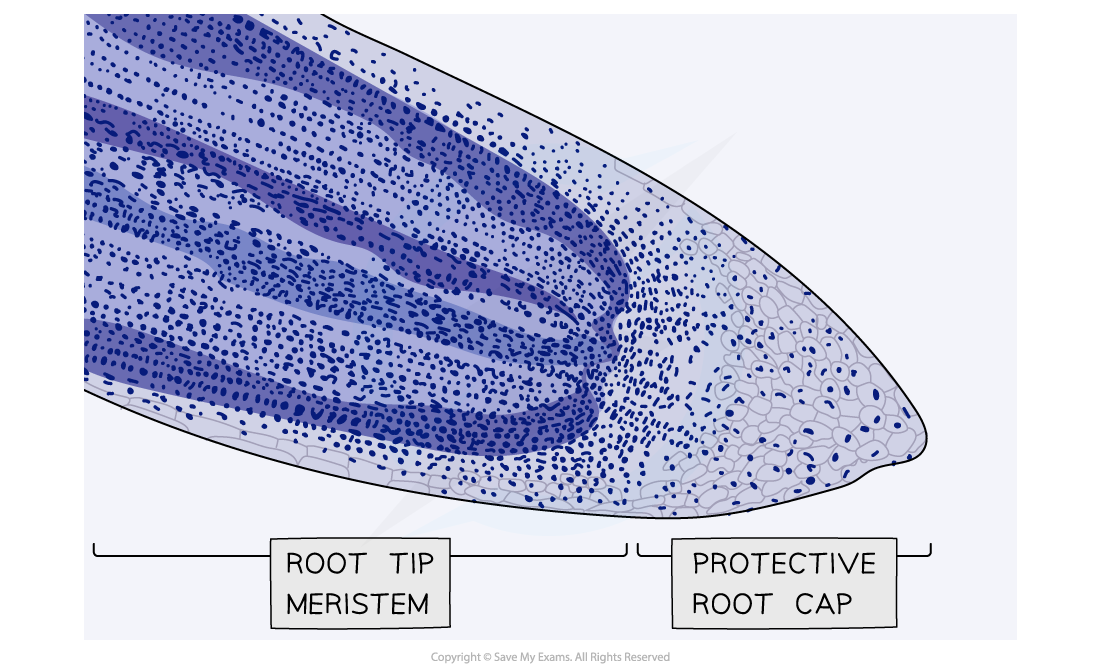# AQA A Level Biology复习笔记2.3.6 Investigating Plant Root Tips

### Maths Skill: Mitotic Index

• The mitotic index is the proportion of cells (in a group of cells or a sample of tissue) that are undergoing mitosis
• The mitotic index can be calculated using the formula below:

mitotic index = number of cells with visible chromosomes ÷ total number of cells

• You can multiply the answer by 100 if you need to give the mitotic index as a percentage

#### Worked Example

A student who wanted to observe mitosis prepared a sample of cells. They counted a total of 42 cells in their sample, 32 of which had visible chromosomes. Calculate the mitotic index for this sample of cells (give your answer to 2 decimal places).

mitotic index = number of cells with visible chromosomes ÷ total number of cells

mitotic index = 32 ÷ 42

mitotic index = 0.76

#### Worked Example

The table below shows the number of cells in different stages of mitosis in a sample from a garlic root tip. Calculate the mitotic index for this tissue (give your answer to 2 decimal places).mitotic index = number of cells with visible chromosomes ÷ total number of cells

mitotic index = (prophase + metaphase + anaphase + telophase) ÷ total number of cells

mitotic index = (14 + 5 + 3 + 6) ÷ (36 + 14 + 5 + 3 + 6)

mitotic index = 28 ÷ 64

mitotic index = 0.44

#### Worked Example

The micrograph below shows a sample of cells from an onion root tip. Calculate the mitotic index for this tissue (give your answer to 2 decimal places).A Sample of cells from an onion root tip

Number of cells with visible chromosomes (green) = 20

Total number of cells (green + red) = 20 + 55 = 75

mitotic index = number of cells with visible chromosomes ÷ total number of cells

mitotic index = 20 ÷ 75

mitotic index = 0.27A sample of cells from an onion root tip

### Maths Skill: Calculating the Size of Root Tip Cells

• Growth in plants occurs in specific regions called meristems
• The root tip meristem can be used to study mitosis
• The root tip meristem can be found just behind the protective root cap
• In the root tip meristem, there is a zone of cell division that contains cells undergoing mitosis
• Pre-prepared slides of root tips can be studied or temporary slides can be prepared using the squash technique (root tips are stained and then gently squashed, spreading the cells out into a thin sheet and allowing individual cells undergoing mitosis to be clearly seen)Micrograph showing a stained root tip

#### Calculating the size of root tip cells

• The images (micrographs) taken of slides containing root tip cells can be used to calculate the actual size of root tip cells
• The magnification equation:

actual size = size of image ÷ magnification

• The size of cells is typically measured using the micrometre (μm) scale, with cellular structures measured in either micrometers (μm) or nanometers (nm)
• When doing calculations all measurements must be in the same units. It is best to use the smallest unit of measurement shown in the question
• To convert units, multiply or divide depending if the units are increasing or decreasing
• Magnification does not have units

#### Exam Tip

• There are 1000 nanometers (nm) in a micrometre (µm)
• There are 1000 micrometres (µm) in a millimetre (mm)
• There are 1000 millimetres (mm) in a metre (m)